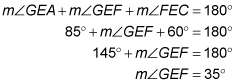##### Geometry: 1,001 Practice Problems For Dummies (+ Free Online Practice)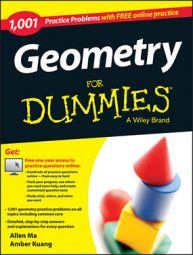When two parallel lines are intersected by a third line — a transversal corresponding pairs of angles are formed. You can use the properties of these angles to find missing angles.

## Practice questions

Use the figures and the given information to solve for the missing angles in the following questions.

1.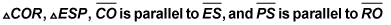as shown in the following figure.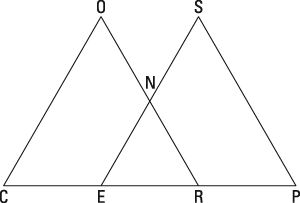If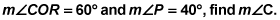2.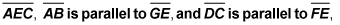as shown in the following figure.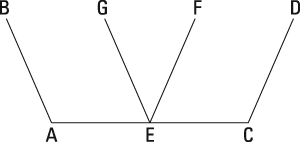If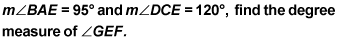## Answers and explanations

1. 80 degrees

When two parallel lines are cut by a transversal, corresponding angles are formed.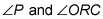are corresponding angles, so they're congruent: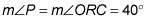The three angles of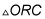add up to 180 degrees, so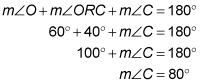2. 35 degrees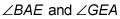are supplementary, which means they add up to 180 degrees: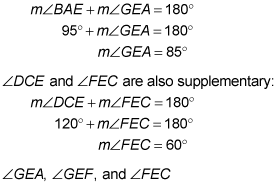form a straight line, so they also add up to 180 degrees: